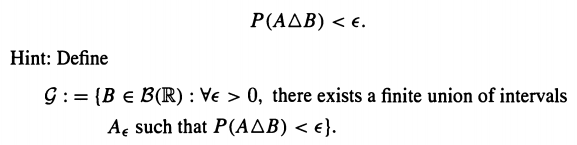### Create an Account

Already have account?

### Forgot Your Password ?

Home / Questions / Let P be a probability measure on ß R For any B B R and any 0 there exists a finite uni...

# Let P be a probability measure on ß R For any B B R and any 0 there exists a finite union of intervals A such that

Let P be a probability measure on ß(R). For any BB(R) and any> 0, there exists a finite union of intervals A such thatJun 22 2020 View more View Less

#### Answer (Solved)Subscribe To Get Solution